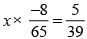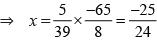Courses

# Olympiad Test: Rational Numbers -2

## 10 Questions MCQ Test Mathematics Olympiad Class 7 | Olympiad Test: Rational Numbers -2

Description
This mock test of Olympiad Test: Rational Numbers -2 for Class 7 helps you for every Class 7 entrance exam. This contains 10 Multiple Choice Questions for Class 7 Olympiad Test: Rational Numbers -2 (mcq) to study with solutions a complete question bank. The solved questions answers in this Olympiad Test: Rational Numbers -2 quiz give you a good mix of easy questions and tough questions. Class 7 students definitely take this Olympiad Test: Rational Numbers -2 exercise for a better result in the exam. You can find other Olympiad Test: Rational Numbers -2 extra questions, long questions & short questions for Class 7 on EduRev as well by searching above.
QUESTION: 1

Solution:
QUESTION: 2

### Which of the following is equivalent to 4/7?

Solution: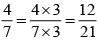QUESTION: 3

### What is the value of x if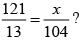Solution: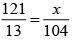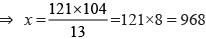QUESTION: 4

What result will be obtained when the sum of 65/12 and 8/3 is divided by their difference?

Solution: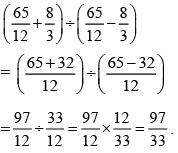QUESTION: 5

​What is multiplicative inverse of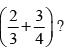Solution: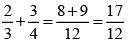Multiplicative inverse of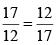QUESTION: 6

The cost of 15 articles is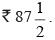What is the cost of one article?

Solution: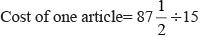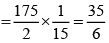QUESTION: 7

A bus is moving at an average speed of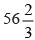km/hour. How much distance it cover in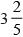hour?

Solution: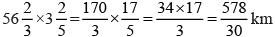QUESTION: 8

What should be added to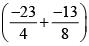to get1?

Solution: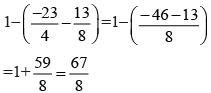QUESTION: 9

How many pieces each of length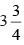m can be cut from a rope of length 60 m?

Solution: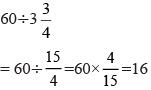QUESTION: 10

By what rational number should –8/65is multiplied to obtain 5/39?

Solution: